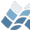NV5 GEOSPATIAL SOFTWARECMCONGRID

CMCONGRID

## Purpose

Shrink or expand the size of an array by an arbitrary amount.
This IDL procedure simulates the action of the VAX/VMS
CONGRID/CONGRIDI function.
This function is similar to "REBIN" in that it can resize a
one, two, or three dimensional array. "REBIN", however,
requires that the new array size must be an integer multiple
of the original size. CONGRID will resize an array to any
arbitrary size (REBIN is somewhat faster, however).
REBIN averages multiple points when shrinking an array,
while CONGRID just resamples the array.

## Category

Array Manipulation.

## Calling Sequence

array = CONGRID(array, x, y, z)

## Inputs

array: A 1, 2, or 3 dimensional array to resize.
Data Type : Any type except string or structure.
x: The new X dimension of the resized array.
Data Type : Int or Long (greater than or equal to 2).

## Optional Inputs

y: The new Y dimension of the resized array. If the original
array has only 1 dimension then y is ignored. If the
original array has 2 or 3 dimensions then y MUST be present.
z: The new Z dimension of the resized array. If the original
array has only 1 or 2 dimensions then z is ignored. If the
original array has 3 dimensions then z MUST be present.

## Keyword Parameters

INTERP: If set, causes linear interpolation to be used.
Otherwise, the nearest-neighbor method is used.
CUBIC: If set, uses "Cubic convolution" interpolation. A more
accurate, but more time-consuming, form of interpolation.
CUBIC has no effect when used with 3 dimensional arrays.
MINUS_ONE:
If set, will prevent CONGRID from extrapolating one row or
column beyond the bounds of the input array. For example,
If the input array has the dimensions (i, j) and the
output array has the dimensions (x, y), then by
default the array is resampled by a factor of (i/x)
in the X direction and (j/y) in the Y direction.
If MINUS_ONE is present (AND IS NON-ZERO) then the array
will be resampled by the factors (i-1)/(x-1) and
(j-1)/(y-1).
HALF_HALF:
If set, will tell CONGRID to extrapolate a *half* row
and column on either side, rather than the default of
one full row/column at the ends of the array. If you
are interpolating images with few rows, then the
output will be more consistent with this technique.
This keyword is intended as a replacement for
MINUS_ONE, and both keywords probably should not be
used in the same call to CONGRID.

## Outputs

The returned array has the same number of dimensions as the original
array and is of the same data type. The returned array will have
the dimensions (x), (x, y), or (x, y, z) depending on how many

## Procedure

IF the input array has three dimensions, or if INTERP is set,
then the IDL interpolate function is used to interpolate the
data values.
If the input array has two dimensions, and INTERP is NOT set,
then the IDL POLY_2D function is used for nearest neighbor sampling.
If the input array has one dimension, and INTERP is NOT set,
then nearest neighbor sampling is used.

## Example

; vol is a 3-D array with the dimensions (80, 100, 57)
; Resize vol to be a (90, 90, 80) array
vol = CONGRID(vol, 90, 90, 80)

## Modification History

DMS, Sept. 1988.
DMS, Added the MINUS_ONE keyword, Sept. 1992.
Daniel Carr. Re-wrote to handle one and three dimensional arrays
using INTERPOLATE function.
DMS, RSI, Nov, 1993. Added CUBIC keyword.
Craig Markwardt, Dec, 1997. Added halfhalf keyword to
more evenly distribute "dead" pixel row
Use uniformly spaced grid points for half_half W. Landsman Feb. 2000
(and slightly modified by C. Markwardt 14 Feb 2000)
Fix in case where INTERP=0 (nearest neighbor interp) and
expanding the image (thanks to Larry Bradley) 28 Mar 2007

© 2023 NV5 Geospatial |  Legal